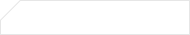# python运算符介绍【python培训】

01. 算数运算符

 运算符 描述 实例 + 加 10 + 20 = 30 - 减 10 - 20 = -10 * 乘 10 * 20 = 200 / 除 10 / 20 = 0.5 // 取整除 返回除法的整数部分（商） 9 // 2 输出结果 4 % 取余数 返回除法的余数 9 % 2 = 1 ** 幂 又称次方、乘方，2 ** 3 = 8

print("-" * 30)02. 比较（关系）运算符

 运算符 描述 == 检查两个操作数的值是否 相等，如果是，则条件成立，返回 True != 检查两个操作数的值是否 不相等，如果是，则条件成立，返回 True > 检查左操作数的值是否 大于 右操作数的值，如果是，则条件成立，返回 True < 检查左操作数的值是否 小于 右操作数的值，如果是，则条件成立，返回 True >= 检查左操作数的值是否 大于或等于 右操作数的值，如果是，则条件成立，返回 True <= 检查左操作数的值是否 小于或等于 右操作数的值，如果是，则条件成立，返回 True

Python 2.x 中判断不等于还可以使用 “<>”运算符

“!=”在 Python 2.x 中同样可以用来判断不等于

03. 逻辑运算符

 运算符 逻辑表达式 描述 and x and y 只有 x 和 y 的值都为 True，才会返回 True 否则只要 x 或者 y 有一个值为 False，就返回 False or x or y 只要 x 或者 y 有一个值为 True，就返回 True 只有 x 和 y 的值都为 False，才会返回 False not not x 如果 x 为 True，返回 False 如果 x 为 False，返回 True

04. 赋值运算符

·在 Python 中，使用 `=` 可以给变量赋值
·在算术运算时，为了简化代码的编写，“Python”还提供了一系列的与算术运算符对应的赋值运算符
·注意：赋值运算符中间不能使用空格

 运算符 描述 实例 = 简单的赋值运算符 c = a + b 将 a + b 的运算结果赋值为 c += 加法赋值运算符 c += a 等效于 c = c + a -= 减法赋值运算符 c -= a 等效于 c = c - a *= 乘法赋值运算符 c *= a 等效于 c = c * a /= 除法赋值运算符 c /= a 等效于 c = c / a //= 取整除赋值运算符 c //= a 等效于 c = c // a %= 取 模 (余数)赋值运算符 c %= a 等效于 c = c % a **= 幂赋值运算符 c **= a 等效于 c = c ** a

05. 运算符的优先级

·以下表格的算数优先级由高到最低顺序排列

 运算符 描述 ** 幂 (最高优先级) * / % // 乘、除、取余数、取整除 + - 加法、减法 <= < > >= 比较运算符 == != 等于运算符 = %= /= //= -= += *= **= 赋值运算符 not or and 逻辑运算符

python是什么?python的用途

Python基础视频教程(600集)

## 相关阅读基础班入门课程限时免费

申请试学名额

基础班入门课程限时免费

申请试学名额

基础班入门课程限时免费

申请试学名额

基础班入门课程限时免费

申请试学名额

基础班入门课程限时免费

申请试学名额

基础班入门课程限时免费

申请试学名额

基础班入门课程限时免费

申请试学名额

基础班入门课程限时免费

申请试学名额

基础班入门课程限时免费

申请试学名额

基础班入门课程限时免费

申请试学名额

基础班入门课程限时免费

申请试学名额

基础班入门课程限时免费

申请试学名额

基础班入门课程限时免费

申请试学名额